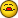vlookup issue

J

John Smith

Hi,
The following code works correctly, except that the vlookup function
is consistently off by three rows. I get all of the correct data in
column P and the data in column S and T is correct, but it all begins
three rows than the data in column P. So the corresponding data at P4
is displayed in cells S7 and T7. What do I need to make it work
correctly?
Thanks.
James

Sub BlanksDumper()
'This sub deletes the blank rows on Sheet 1, column P and
' retrieves data using the individual's ID number
Application.ScreenUpdating = False
Dim DataRng As Range
Dim r As Integer
Dim LastCellRowNumber As Long

Sheets("Sheet1").Select

LastRow = Cells(Rows.Count, "P").End(xlUp).Row
Set DataRng = Range("P4" & LastRow)

For r = LastRow To 4 Step -1
If DataRng(r) = "" Then
DataRng(r).EntireRow.Delete
Else
DataRng(r).Offset(0, 3).Value = "=VLOOKUP(K" & r & " ,
Beg_to_S1, 5, False)" DataRng(r).Offset(0, 4).Value =
"=VLOOKUP(K" & r & " , Beg_to_S1, 4, False)" End If
Next r

Set DataRng = Nothing
Application.ScreenUpdating = True
LastCellRowNumber = Range("P" & Rows.Count).End(xlUp).Row
End Sub

T

Tim Williams

You 'DataRng' range doesn't have 'LastRow' number of cells in it,
since it doesn't start on the first row, but on row 4.

DataRng(4) is actually on row 7

Tim

J

John Smith

You 'DataRng' range doesn't have 'LastRow' number of cells in it,
since it doesn't start on the first row, but on row 4.

DataRng(4) is actually on row 7

Tim

- Show quoted text -

You are correct, Tim! Thank you, it works great now.
James